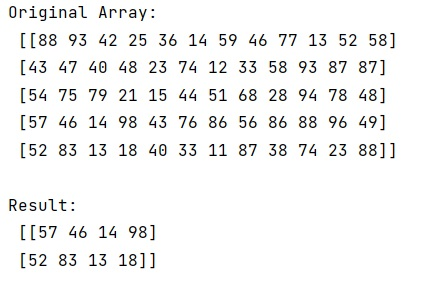# How to crop center portion of a NumPy image?

Python | numpy.polyfit(): Learn about the numpy.polyfit() method, its usages and example.
Submitted by Pranit Sharma, on February 27, 2023

NumPy is an abbreviated form of Numerical Python. It is used for different types of scientific operations in python. Numpy is a vast library in python which is used for almost every kind of scientific or mathematical operation. It is itself an array which is a collection of various methods and functions for processing the arrays.

## Cropping center portion of a NumPy image

As we know a picture contains pixels arranged as a matrix hence, we can import the pixels of this image as a matrix.

Suppose that we are given a numpy image of some width x and height y. I have to crop the center portion of the image to width x and height y. We are assuming that x and y are positive non-zero integers and less than the respective image size.

For this purpose, we will define a function inside which we just have to slice the image along the points we will define as x and y.

Let us understand with the help of an example,

## Python code to crop center portion of a NumPy image

```# Import numpy
import numpy as np

# Creating a numpy image
arr = np.array([[88, 93, 42, 25, 36, 14, 59, 46, 77, 13, 52, 58],
[43, 47, 40, 48, 23, 74, 12, 33, 58, 93, 87, 87],
[54, 75, 79, 21, 15, 44, 51, 68, 28, 94, 78, 48],
[57, 46, 14, 98, 43, 76, 86, 56, 86, 88, 96, 49],
[52, 83, 13, 18, 40, 33, 11, 87, 38, 74, 23, 88]])

# Display original image
print("Original Array:\n",arr,"\n")

# Defining a function
def fun(i,cx,cy):
x,y = i.shape
startx = x // 2 - (cx // 2)
starty = y // 2 - (cy // 2)
return i[starty:starty+cy,startx:startx+cx]

# Display result
print("Result:\n",fun(arr,4,6))
```

Output:Languages: » C » C++ » C++ STL » Java » Data Structure » C#.Net » Android » Kotlin » SQL
Web Technologies: » PHP » Python » JavaScript » CSS » Ajax » Node.js » Web programming/HTML
Solved programs: » C » C++ » DS » Java » C#
Aptitude que. & ans.: » C » C++ » Java » DBMS
Interview que. & ans.: » C » Embedded C » Java » SEO » HR
CS Subjects: » CS Basics » O.S. » Networks » DBMS » Embedded Systems » Cloud Computing
» Machine learning » CS Organizations » Linux » DOS
More: » Articles » Puzzles » News/Updates